## Developer Insights: Arena Balance Through Science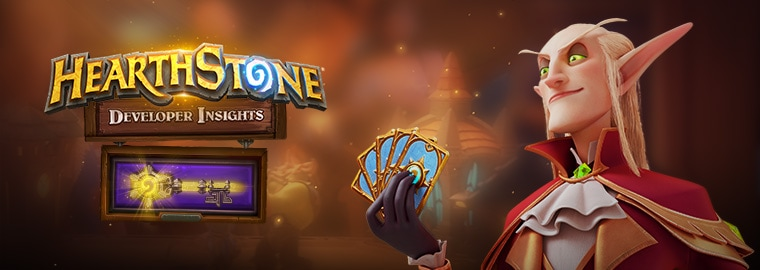Hi! I’m Tian, a Senior Data Scientist with the Hearthstone team, and today we’re talking about the math behind Arena balance.

Arena matches are played all the time, and they constantly generate data—A LOT of data—that we can use to help make sure that Arena is more balanced. If you had to place me in Boom Labs, I’d probably be in the Mathematical Science department!

#### The Balancing Game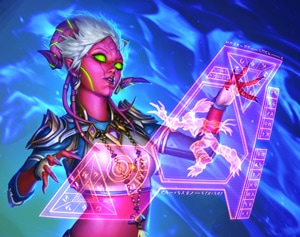Arena balance is done in two stages. First, we determine which bucket(s) each card goes into (a bucket is a subset of cards with similar performance quality). A card generally falls into two buckets, and we divide Legendary and non-Legendary cards into two different bucket systems. We decide which bucket each card falls into by its win-rate and pick-rate during games. That means each of the three cards you see during a pick are on similar power tiers.

We then balance the win-rate across the nine classes. Ideally, it’s as close to 50% as possible. We achieve this balance by tuning the weights associated with each card. Weight is a number that represents the relative likelihood that a card appears in a draft. The more weight a card has, the higher the chance that you’ll see it during a draft. If the weight of a card is changed, that also changes the chances that the bucket it’s in will appear.

A lot of data is needed to make the system work, but due to the huge number of Arena games played daily, we have plenty of data to utilize.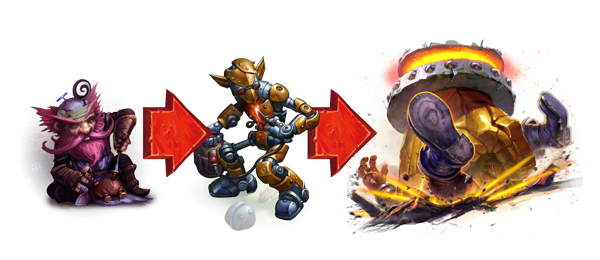Utilizing that data to affect game balance requires three steps.

1. Build a model
2. Solve constrained optimization problems
3. Calculate the weights

After all that’s done, we need to schedule hotfixes to apply the changes.

#### Build A Model

If you’re a frequent Arena player, you might be familiar with calculating win probability. Some cards skew probability more heavily than others. For example, drawing The Lich King in a game would affect your win probability a lot more than drawing Snowflipper Penguin.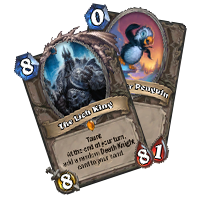Let’s assume you draw The Lich King during a game. You may start thinking: “What is my win probability now that I’ve drawn The Lich King? Is it 60%? 50%? How do I evaluate this quantitatively?” Further assume that you draw Ice Barrier on your next turn—now you’ll want to re-evaluate your win probability once more.

We built a machine learning model to answer those questions. The computer is fed tons of data; using details from every Arena game played, it learns how to predict the win probability based on all the information it has access to. In slightly more formal terms, we “train” the model that we built. Thus, it’s able to provide the answer on win probability given X cards drawn every time we ask.

#### Solving Constrained Optimization Problems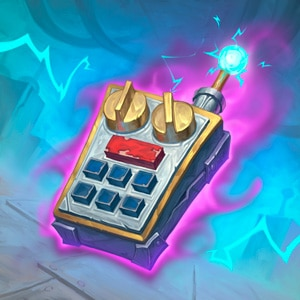Let’s take a step back and imagine that the model is a box with lots of knobs that you can tune. Each knob is associated with a specific card. When you tune a knob, you are in fact tuning numbers associated with that card.

Let’s say that before you tune a knob, the box tells you that the current win probability is 40%. After you make a turn, the predicted win probability changes to 46%. This poses a very interesting question: if you tune a bunch of knobs, will you be able to turn the win-probability to something you desire?

This question leads to the idea that we need to construct an optimization problem. In mathematical terms, we want to find the best solution from all feasible solutions. We want to make a target as close as possible to what we want by “tuning a bunch of knobs” at the same time. In formal terms, we minimize some objective function over a high-dimensional vector.

In Arena balance, we want the predicted win-rate to be as close as possible to 50% regardless of class, and we change numbers associated with each card to achieve that.

However, the knobs can’t be tuned arbitrarily—some constraints apply. Here’s a list of some constraints we have programmed into our “box”.

• The new number should be within +/-30% range of some fixed value. Drastic changes can potentially harm the gameplay experience.
• If we want to reduce the power of a class in Arena, its strongest cards will need to appear less often than its less powerful cards. Vice versa if we want a class to get stronger.
• There are some hard constraints required to keep the problem valid. For example, the total gains in the appearance probability need to be the same as the total losses (zero-sum, in mathematical terms)

#### Calculate the Weights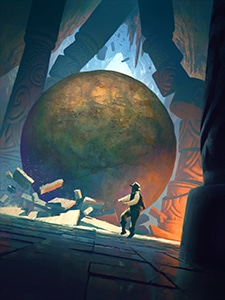The final step in using Arena data to affect balance is to adjust the weights assigned to each card based on what we get from the first two steps. In general, a card with a weight of 2.0 shows up twice as often as a card with a weight of 1.0. The constrained optimization tells us which “knobs” to tune and how much to tune these knobs. We then link each knob to the probability of each card showing up in a draft. Now we know how much we need to change the weight of each card, not counting other modifiers derived from the card’s traits (e.g., whether it’s a spell or weapon, which expansion it’s from, etc.)

#### Leveling the Playing Field

After this stage of the balance is done, the overall win-rate across all nine classes should be very close to +/-50%. However, there have been rare situations where the win-rate after balance is still not ideal. That can happen if a certain class' win rate is too far away from 50% before we do the weight adjustments. In those cases we might not hit our ideal numbers, but they’ll still be in better shape than what they were before.

Thanks to this system being able to utilize Arena data in advanced computational mathematics and machine learning, we’re able to determine whether a class needs to be strengthened or weakened, and then choose the optimal weight for each card for each class.I hope this insight into our micro-adjustment system for Arena was interesting! We want to know what you think, so please let us know if you have any questions in the comments